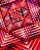# Six terms

Find the first six terms of the sequence
a1 = -3, an = 2 * an-1

a1 =  -3
a2 =  -6
a3 =  -12
a4 =  -24
a5 =  -48
a6 =  -96

### Step-by-step explanation:

${a}_{1}=-3$We will be pleased if You send us any improvements to this math problem. Thank you!## Related math problems and questions:

• 6 termsFind the first six terms of the sequence. a1 = 7, an = an-1 + 6
• Five elementThe geometric sequence is given by quotient q = 1/2 and the sum of the first six members S6 = 63. Find the fifth element a5.
• Sum 1-6Find the sum of the geometric progression 3, 15, 75,… to six terms.
• Sequence 11What is the nth term of this sequence 1, 1/2, 1/3, 1/4, 1/5?
• Geometric sequence 4It is given geometric sequence a3 = 7 and a12 = 3. Calculate s23 (= sum of the first 23 members of the sequence).
• Geometric progression 2There is geometric sequence with a1=5.7 and quotient q=-2.5. Calculate a17.
• Five membersWrite first 5 members geometric sequence and determine whether it is increasing or decreasing: a1 = 3 q = -2
• GP - three membersThe second and third of a geometric progression are 24 and 12(c+1), respectively, given that the sum of the first three terms of progression is 76. determine the value of c.
• GP membersThe geometric sequence has 10 members. The last two members are 2 and -1. Which member is -1/16?
• SequenceFind the common ratio of the sequence -3, -1.5, -0.75, -0.375, -0.1875. Ratio write as decimal number rounded to tenth.
• Find the 21Find the sum of the six terms of the finite geometric sequence 96, -48, 24, -12
• Sequence 2Write the first 5 members of an arithmetic sequence a11=-14, d=-1
• Geometric sequence 3In geometric sequence is a8 = 312500; a11= 39062500; sn=1953124. Calculate the first item a1, quotient q, and n - number of members by their sum s_n.
• Geometric sequenceIn the geometric sequence is a4 = 20 a9 = -160. Calculate the first member a1 and quotient q.
• Sequence 3Write the first 5 members of an arithmetic sequence: a4=-35, a11=-105.
• Arithmetic progression 2The 3rd term of an Arithmetic progression is ten more than the first term, while the fifth term is 15 more than the second term. Find the sum of the 8th and 15th terms of the Arithmetic progression if the 7th term is seven times the first term.
• AP - simpleFind the first ten members of the sequence if a11 = 132, d = 3.